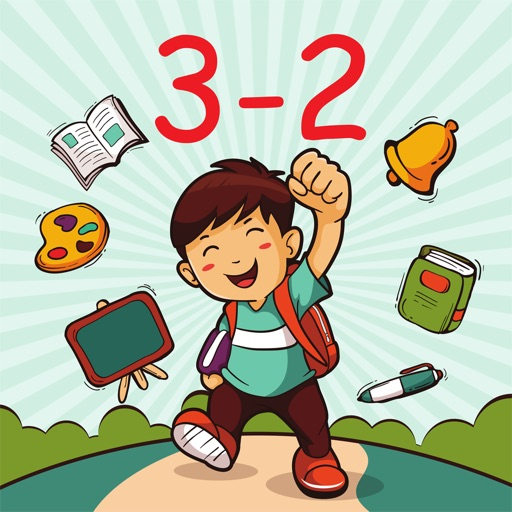more ▼## Subtraction Math TrainerClick here to request a review of this app

• App Store Info

### Description

Subtraction Math Trainer is a math learning app designed to help you to practice subtraction. The built-in handwriting recognition allows you to draw answers directly on the screen. Comes with a number of simple mini-games to make learning more effective and less boring.

You can practice the following math skills:

Subtraction facts - numbers up to 10
Subtraction facts - numbers up to 18
Subtract a multiple of ten
Subtraction facts - numbers up to 20
Subtract a one-digit number from a two-digit number
Subtract two two-digit numbers
Subtract multiples of 10
Subtract multiples of 100
Subtract multiples of 10 or 100
Balance subtraction equations
Subtraction facts - numbers up to 100
Subtract two three-digit numbers
Complete the subtraction sentence up to three digits
Subtract numbers with four or five digits
Balance subtraction equations up to three digits

### What's New in Version 2.0.0

New design. Extended selection of math skills to practice.